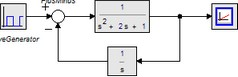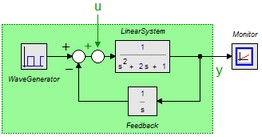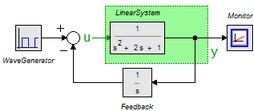﻿ 20-sim webhelp > Toolboxes > Frequency Domain Toolbox > Model Linearization > Linearization Explained

# Linearization Explained

Navigation:  Toolboxes > Frequency Domain Toolbox > Model Linearization >

# Linearization ExplainedDuring linearization you are asked to enter an input signal and an output signal of your (non-linear) model. During linearization, 20-sim will derive a corresponding linear system. It is important to understand that 20-sim will cut the model at the input. This will be explained with the example model below. It shows a linear system with feedback.## Closed Loop

Suppose we would choose the following settings:

input u: WaveGenerator\output

output y: Monitor\input

kind: Closed Loop

This means that 20-sim will add an input u and linearize the model between this variable and the variable Monitor\output (equal to y in the figure below). 20-sim will thus linearize the system between u and y and (in this example) yield the closed loop system.## Open Loop

Suppose we would choose the following settings:

input u: PlusMinus\output

output y: Monitor\input

kind: Open Loop

This means that 20-sim will cut the model at the variable PlusMinus\output (equal to u in the figure below) and linearize the model between this variable and the variable Monitor\input (equal to y in the figure below). 20-sim will thus linearize the system between u and y and (in this example) yield the open loop system.